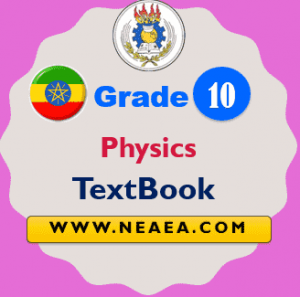# Ethiopian Grade 10 Physics Textbook for Students [PDF]

Ethiopian Grade 10 Chemistry Textbook For Students [PDF] Download: The grade 10 student can study this Physics book for better national exam preparation. It is provided by the MoE Ethiopia.

Understand the concepts of, electrical, gravitational, and magnetic fields; electromagnetic radiation; electromagnetic induction, and the interface between energy and matter, the common applications of electrical and electronic circuits, and the function and configuration of the components used in circuits.## Ethiopian Grade 10 Physics Student Textbook

This textbook will help students to develop skills in using measuring instruments and familiar electrical devices, constructing simple electrical circuits using common tools appropriately and safely and appreciate the applications of electrical and electronic technologies to the community

Unit 1: Motion in two dimensions

• 1.1. Projectile motion
• 1.2. Rotational kinematics
• 1.3. Rotational dynamics
• 1.4. Newton’s law of universal gravitation

Unit 2: Electrostatics

• 2.1. Electric charges
• 2.2. Electric forces and fields
• 2.3. Electric potential
• 2.4. Capacitors and capacitance

Unit 3: Current electricity

• 3.1. Electric current
• 3.2. Ohm’s law and electrical resistance
• 3.3. Combinations of resistors
• 3.4. Emf and internal resistance of a cell
• 3.5. Electric energy and power
• 3.6. Electric installation and safety rules

Unit 4: Electromagnetism

• 4.1. Magnetism
• 4.2. Concepts of magnetic field
• 4.3. Magnetic force
• 4.4. Electromagnetic induction

Unit 5: Electronics

• 5.1. Vacuum tube devices
• 5.2. Conductors, semiconductors and insulators
• 5.3. Semiconductors
• 5.4. Transistors

Unit 6: Electromagnetic waves and geometrical optics

• 6.1. Electromagnetic waves
• 6.2. Reflection of light
• 6.3. Refraction of light

### Ethiopian Grade 10 Physics Textbook [PDF]

After completing grade 10 Physics Textbook lessons the students will be able to understand the motion of objects in horizontal, vertical, and inclined planes, and with reference to the forces acting on the objects; the laws of conservation of energy and of momentum for objects moving in one and two dimensions.